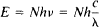# Einstein Law of Photochemical Equivalence

The following article is from The Great Soviet Encyclopedia (1979). It might be outdated or ideologically biased.

## Einstein Law of Photochemical Equivalence

a fundamental law of photochemistry that establishes that every photon absorbed causes one elementary reaction. The reaction may consist in the chemical transformation of molecules of the substance or in their physical excitation and the emission of the energy absorbed (or in the transformation of the energy into thermal energy). The number N of reacted molecules is related to the energy E absorbed by the system by the equationwhere v is the frequency of radiation, c is the velocity of light, λ is the wavelength of the light, and h is Planck’s constant. The quantity γ (the quantum yield of the photochemical reaction), which is equal to the ratio of the number of reacted molecules of a given substance to the number of light quanta absorbed, is usually the criterion for applicability of the Einstein law of photochemical equivalence. According to the law, γ must be equal to unity.

The deviations from the Einstein law of photochemical equivalence that are frequently observed in nature are usually explained by secondary processes (for more details seePHOTOCHEMISTRY). The law was discovered in 1912 by Albert Einstein.